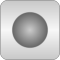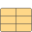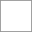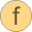# R134a_ph

Medium model for R134a and p,h as states# Package ContentsSaturationPropertiesThermodynamicState Thermodynamic stateBaseProperties Base properties of R134asetState_phX Set state for pressure and specific enthalpy (X not used since single substance)setState_dTX Set state for density and temperature (X not used since single substance)setState_psX Set state for pressure and specific entropy (X not used since single substance)setState_pTX Set state for pressure and temperature (X not used since single substance)setBubbleState Return the thermodynamic state on the bubble linesetDewState Return the thermodynamic state on the dew linedensity_ph Density as function of pressure and specific enthalpydensity Density as function of pressure and specific enthalpy | use setState_phX function for inputtemperature_ph Temperature as function of pressure and specific enthalpytemperature Temperature as function of pressure and specific enthalpy | use setState_phX function for inputpressure Pressure w.r.t. thermodynamic statespecificInternalEnergy Specific internal energy w.r.t. thermodynamic statespecificEnthalpy Specific enthalpy w.r.t. thermodynamic state | use setState_phX function for inputspecificEntropy Specific entropy w.r.t. thermodynamic state | use setState_phX function for input if necessarysaturationTemperature Saturation temperature in two-phase regionsaturationTemperature_derp Derivative of saturation temperature in two-phase regionsaturationTemperature_der_p Time derivative of saturation temperature in two-phase regionbubbleDensity Density of liquid phase w.r.t saturation pressure | use setSat_p function for inputdBubbleDensity_dPressure Derivative of liquid density in two-phase region w.r.t pressuredBubbleDensity_dPressure_der_sat Time derivative of liquid density in two-phase region w.r.t pressuredewDensity Density of vapor phase w.r.t saturation pressure | use setSat_p function for inputdDewDensity_dPressure Derivative of vapor density in two-phase region w.r.t pressuredDewDensity_dPressure_der_sat Time derivative of vapor density in two-phase region w.r.t pressurebubbleEnthalpy Specific enthalpy of liquid phase w.r.t saturation pressure | use setSat_p function for inputdBubbleEnthalpy_dPressure Derivative of liquid specific enthalpy in two-phase region w.r.t pressuredBubbleEnthalpy_dPressure_der_sat Time derivative of liquid specific enthalpy in two-phase region w.r.t pressuredewEnthalpy Specific enthalpy of vapor phase w.r.t saturation pressure | use setSat_p function for inputdDewEnthalpy_dPressure Derivative of vapor specific enthalpy in two-phase region w.r.t pressuredDewEnthalpy_dPressure_der_sat Time derivative of vapor specific enthalpy in two-phase region w.r.t pressuredewEntropy Specific entropy of vapor phase w.r.t saturation pressure | use setSat_p function for inputdDewEntropy_dPressure Derivative of vapor specific entropy in two-phase region w.r.t pressure | use setState_phX function for inputdDewEntropy_dPressure_der_sat Time derivative of vapor specific entropy in two-phase region w.r.t pressure | use setState_phX function for inputbubbleEntropy Specific entropy of liquid phase w.r.t saturation pressure | use setSat_p function for inputdBubbleEntropy_dPressure Derivative of liquid specific entropy in two-phase region w.r.t pressure | use setState_phX function for inputdBubbleEntropy_dPressure_der_sat Time derivative of liquid specific entropy in two-phase region w.r.t pressure | use setState_phX function for inputsaturationPressure Saturation pressure w.r.t. temperaturespecificHeatCapacityCp Specific heat capacity at constant pressure | turns infinite in two-phase region! | use setState_phX function for inputspecificHeatCapacityCv Specific heat capacity at constant volume | use setState_phX function for inputdynamicViscosity Dynamic viscosity w.r.t. temperature and density | use setState_phX function for inputthermalConductivity Thermal conductivity w.r.t. thermodynamic state | use setState_phX function for inputsurfaceTension Surface tension as a function of temperature (below critical point)velocityOfSound Velocity of sound w.r.t. thermodynamic state (only valid for one-phase)isothermalCompressibility Isothermal compressibility w.r.t. thermodynamic state (only valid for one-phase)isobaricExpansionCoefficient Isobaric expansion coefficient w.r.t. thermodynamic state (only valid for one-phase)isentropicExponent Isentropic exponent gamma w.r.t. thermodynamic state | not defined in two-phase region | use setState_phX function for inputspecificGibbsEnergy Specific gibbs energy w.r.t. thermodynamic statespecificHelmholtzEnergy Helmholtz energy w.r.t. thermodynamic statedensity_derh_p Density derivative by specific enthalpy | use setState_phX function for inputdensity_derp_h Density derivative by pressure | use setState_phX function for inputisentropicEnthalpy Isentropic enthalpy of downstream pressure and upstream thermodynamic state (specific entropy)derivsOf_ph Derivatives required for inversion of temperature and density functionsdt_ph Density and temperature w.r.t. pressure and specific enthalpydtofphOnePhase Density and temperature w.r.t. pressure and specific enthalpy in one-phase regiondtofpsOnePhase Inverse iteration in one phase region (d,T) = f(p,s)f_R134a Calculation of helmholtz derivatives by density and temperaturefid_R134a Helmholtz coefficients of ideal partfres_R134a Calculation of helmholtz derivativesgetPhase_ph Number of phases by pressure and specific enthalpygetPhase_ps Number of phases by pressure and entropyhofpsTwoPhase Isentropic specific enthalpy in two phase region h(p,s)R134a_liqofdT Properties on liquid boundary phaseR134a_vapofdT Properties on vapor boundary phaserho_ph_der Time derivative function of density_phrho_props_ph Density as function of pressure and specific enthalpyT_ph_der Time derivative function of T_phT_props_ph Temperature as function of pressure and specific enthalpysetSmoothState Smooth transition function between state_a and state_bdofpT Compute d for given p and ThofpT Compute h for given p and TphaseBoundaryAssert Assert function for checking threshold to phase boundary

# Package Constants (27)

ThermoStates Value: Modelica.Media.Interfaces.Choices.IndependentVariables.ph Type: IndependentVariables Description: Enumeration type for independent variables Value: "R134a_ph" Type: String Description: Name of the medium Value: {"tetrafluoroethan"} Type: String[:] Description: Names of the mixture substances. Set substanceNames={mediumName} if only one substance. Value: fill("", 0) Type: String[:] Description: Names of the additional (extra) transported properties. Set extraPropertiesNames=fill("",0) if unused Value: false Type: Boolean Description: = true, if u and d are not a function of pressure Value: true Type: Boolean Description: = true if medium contains the equation sum(X) = 1.0; set reducedX=true if only one substance (see docu for details) Value: true Type: Boolean Description: = true if medium contains the equation X = reference_X Value: 101325 Type: AbsolutePressure (Pa) Description: Reference pressure of Medium: default 1 atmosphere Value: 298.15 Type: Temperature (K) Description: Reference temperature of Medium: default 25 deg Celsius Value: fill(1 / nX, nX) Type: MassFraction[nX] (kg/kg) Description: Default mass fractions of medium Value: 101325 Type: AbsolutePressure (Pa) Description: Default value for pressure of medium (for initialization) Value: Modelica.SIunits.Conversions.from_degC(20) Type: Temperature (K) Description: Default value for temperature of medium (for initialization) Value: 420e3 Type: SpecificEnthalpy (J/kg) Description: Default value for specific enthalpy of medium (for initialization) Value: reference_X Type: MassFraction[nX] (kg/kg) Description: Default value for mass fractions of medium (for initialization) Value: fill(0, nC) Type: ExtraProperty[nC] Description: Default value for trace substances of medium (for initialization) Value: size(substanceNames, 1) Type: Integer Description: Number of substances Value: nS Type: Integer Description: Number of mass fractions Value: if fixedX then 0 else if reducedX then nS - 1 else nS Type: Integer Description: Number of structurally independent mass fractions (see docu for details) Value: size(extraPropertiesNames, 1) Type: Integer Description: Number of extra (outside of standard mass-balance) transported properties Value: 1.0e-6 * ones(nC) Type: Real[nC] Description: Default for the nominal values for the extra properties Value: false Type: Boolean Description: True if the (derived) model should not generate state events Value: false Type: Boolean Description: True if the (derived) model should never be called with two-phase inputs Value: r134aConstants Type: FluidConstants[nS] Description: Constant data for the fluid Value: true Type: Boolean Value: false Type: Boolean Value: Type: FluidLimits Value: Type: FluidConstants

# Information

This information is part of the Modelica Standard Library maintained by the Modelica Association.

Calculation of fluid properties for Tetrafluoroethane (R134a) in the fluid region of 0.0039 bar (Triple pressure) to 700 bar and 169.85 Kelvin (Triple temperature) to 455 Kelvin.

#### Restriction

The functions provided by this package shall be used inside of the restricted limits according to the referenced literature.

• 0.0039 bar ≤ p ≤ 700 bar
• 169.85 Kelvin ≤ T ≤ 455 Kelvin
• explicit for pressure and specific enthalpy

References

Baehr, H.D. and Tillner-Roth, R.:
Thermodynamic Properties of Environmentally Acceptable Refrigerants - Equations of State and Tables for Ammonia, R22, R134a, R152a, and R123. Springer-Verlag, Berlin (Germany), 1994.
Klein, McLinden and Laesecke:
An improved extended corresponding states method for estimation of viscosity of pure refrigerants and mixtures. Int. J. Refrig., Vol. 20, No.3, pp. 208-217, 1997.
McLinden, Klein. and Perkins:
An extended corresponding states model for the thermal conductivity of refrigerants and refrigerant mixtures. Int. J. Refrig., 23 (2000) 43-63.